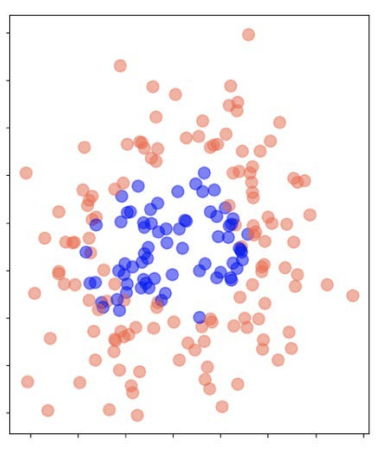시간 제한메모리 제한제출정답맞힌 사람정답 비율
1 초 512 MB42022319252.316%

## 문제

The strike zone in baseball is the volume of space which a baseball must pass through in order to be called a strike, if the batter does not swing. A baseball that misses the strike zone is called a ball, if the batter does not swing. Figure H.1 shows the locations of baseballs at plate which were captured by a ball tracking device during a baseball match. Each blue point was called a strike and each red point was called a ball during the match. This may motivate us to define a rectangular region that represents the strike zone of the match, by analyzing such a ball tracking data of the match.Figure H.1: The locations of baseballs at plate during a baseball match. Blue points were called strikes and red points were called balls.

In this problem, you are given two sets, P1 and P2, of points in the plane and two positive constants c1 and c2. You are asked to find an axis-parallel rectangle R that maximizes the evaluation function eval(R) = c1 × s - c2 × b, where s is the number of points in P1R and b is the number of points in P2R.

## 입력

Your program is to read from standard input. The input starts with a line containing an integer n1 (1 ≤ n1 ≤ 1,000), where n1 denotes the number of points in P1. In the following n1 lines, each line consists of two integers, ranging -109 to 109, representing the coordinates of a point in P1. The next line contains an integer n2 (1 ≤ n2 ≤ 1,000), where n2 denotes the number of points in P2. In the following n2 lines, each line consists of two integers, ranging -109 to 109, representing the coordinates of a point in P2. There are no two points in P1P2 that share the same x or y coordinate. Then the next line consists of two integers, c1 and c2, ranging 1 to 10,000.

## 출력

Your program is to write to standard output. Print exactly one line consisting of one integer that is eval(ܴR), where R is an axis-parallel rectangle with the maximum possible eval value for P1 and P2 with respect to c1 and c2.

## 예제 입력 1

2
-1 -1
4 4
2
0 0
2 2
5 2


## 예제 출력 1

6


## 예제 입력 2

3
0 5
3 3
8 -1
3
1 4
6 0
7 1
3 2


## 예제 출력 2

4